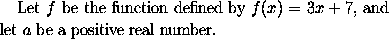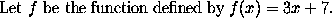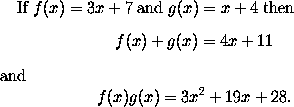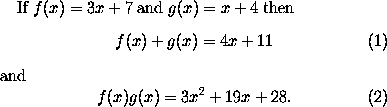### Mathematics Mode

In order to obtain a mathematical formula using LaTeX, one must enter mathematics mode before the formula and leave it afterwards. Mathematical formulae can occur either embedded in text or else displayed between lines of text. When a formula occurs within the text of a paragraph one should place a $ sign before and after the formula, in order to enter and leave mathematics mode. Thus to obtain a sentence likeone should type Let$f$be the function defined by$f(x) = 3x + 7$, and let$a$be a positive real number.  In particular, note that even mathematical expressions consisting of a single character, like f and a in the example above, are placed within $ signs. This is to ensure that they are set in italic type, as is customary in mathematical typesetting.

LaTeX also allows you to use $$ and $$ to mark the beginning and the end respectively of a mathematical formula embedded in text. Thusmay be produced by typing
Let $$f$$ be the function defined by $$f(x) = 3x + 7$$.

However this use of $$ ... $$ is only permitted in LaTeX: other dialects of TeX such as Plain TeX and AmSTeX use $ ... $.

In order to obtain an mathematical formula or equation which is displayed on a line by itself, one places $ before and $ after the formula. Thus to obtainone would type
If $f(x) = 3x + 7$ and $g(x) = x + 4$ then
$f(x) + g(x) = 4x + 11$
and
$f(x)g(x) = 3x^2 + 19x +28.$

(Here the character ^ is used to obtain a superscript.)

LaTeX provides facilities for the automatic numbering of displayed equations. If you want an numbered equation then you use \begin{equation} and \end{equation} instead of using $ and $ . Thus

If $f(x) = 3x + 7$ and $g(x) = x + 4$ then
\begin{equation}
f(x) + g(x) = 4x + 11
\end{equation}
and
\begin{equation}
f(x)g(x) = 3x^2 + 19x +28.
\end{equation}

produces